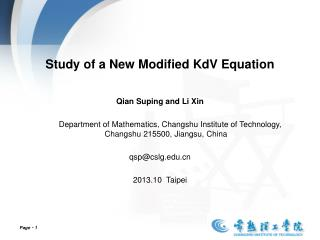DownloadDownload PresentationPage  1

# Page  1

Download Presentation## Page  1

- - - - - - - - - - - - - - - - - - - - - - - - - - - E N D - - - - - - - - - - - - - - - - - - - - - - - - - - -
##### Presentation Transcript

1. Study of a New Modified KdV Equation Qian Suping and Li Xin Department of Mathematics, Changshu Institute of Technology, Changshu 215500, Jiangsu, China qsp@cslg.edu.cn 2013.10 Taipei Page 1

2. Outline Introduction The relation between mKdV and NMKdV Recursion and Inverse Recursion Operators Group invariant solutions Summary and discussions Page 2

3. Introduction The mKdV equation (1) is one of the most important nonlinear systems and a diversity of nonlinear physical phenomena can be successively explained by this simple model. Recently, to describe the interactions between KdV solitons and cnoidal periodic waves, Lou proposed a new modified KdV (NMKdV) equation (2) which is a combination of the potential mKdV equation (without the second term of (2) and the Schwarzian KdV equation (without the third term of (2)). Page 3

4. We investigate NMKdV’s 1. Integrable properties Transformation relation between mKdV andNMKdV Recursion operator and its inverse Infinitely many symmetries (Local and Nonlocal) 2. Exact solutions Symmetry reductions Painleve II reduction Jacobi elliptic function reduction Interactions between soliton and cnoidal periodic waves Page 4

5. The relation between mKdV and NMKdV For the mKdV equation (1), the generalized truncated tanh function expansion canhave the form (3) where u0, u1 and v are arbitrary functions of {x, t} and should be determined later.The usual tanh function expansion method with u0 and u1 being constants and v = kx + ωtis used to find the single kink soliton solution of the mKdV equation.Substituting (3) into (1) we have (4) Page 5

6. Vanishing the coefficients of tanhi(v) for i = 4, 3, 2, we have (5) (6) Substituting (5) and (6)into (4), the remained items became Substituting (5) into (3), we have (7) Page 6

7. Recursion and Inverse Recursion Operators For the mKdV equation (1) and NMKdV (2), the symmetry definition equations read and respectively.From the transformation relation (7), the symmetry relation It is known that for a (1+1)-dimensional integrable system, there exist a recursion operatorΦ, such that a set of infinitely many symmetries can be obtained by acting the recursion operator on a seed symmetry σ0 (8) Page 7

8. From the symmetry transformation relation (3.5), we can find the relation between the recursionoperators Φ (the recursion operator of the mKdV equation) and (the recursion operatorof the NMKdV) One recursion operator of the mKdV give out the factor forms of Φ and T: Using the above results, we get the recursion operator and the inverse recursion operator of the NMKdV (2) as followed: Page 8

9. using thestandard classical Lie group approach or the standard classical Lie symmetry approach,one can find that the only possible Lie point symmetries of the NMKdV (2) are the symmetriesrelated to Clearly, N0(−1) can be obtained from N0(1)by using the discreteinvariant transformation v → −vof (2) and can be recombined as the seeds, Page 9

10. and The symmetry N0(0)≡ 1 is related to the field v translation invariance of (2). Applying the recursion operator Φ to the seeds K0, τ0 and N0(γ), we can find five sets ofinfinitely many general symmetries, the K symmetries, τ symmetries, and N(γ) symmetries Page 10

11. For the Kn symmetries, n ≥ 0 because of K0 is a kernel of the recursion operator. Similarly,n ≥ 0 for Nn(γ)symmetries because of N0(γ)are all kernels of the inverse recursion operator −1. It is not difficult to find that the Kn symmetries for n ≥ 0, Nn(γ) and τn symmetries for n = 0are local symmetries while all others are nonlocal ones. Page 11

12. 不需要局域化，或者说 已经局域化、点李对称化 Group invariant solutions To looking for the group invariant solutions under the symmetry algebra, we have to solvethe symmetry constraint condition (9) Solving the symmetry constraint condition (9), we have two important cases. Case I: c3≠0. Forc3≠0, the general solution of the symmetry constraint condition (9) hasthe form Page 12

13. (10) Substituting (10) into (2), it is straightforward to find the similarity reduction for theundetermined group invariant function which is a variant form of the Painleve II transcendent that means the solution (10) is aninteraction solution between one soliton and the Painlev´e II wave for the NMKdV (2). Page 13

14. Case II: c3 = 0. In this case, the general solution of the group invariant condition (9) reads (11) The group invariant solution for the field Q = Q(η) ≡ Pη can be obtained after substituting(11) into (2). The result reads (12) with C1 being an arbitrary integrating constant. Page 14

15. Obviously, the solution of (12) can be expressed by means of the Jacobi elliptic functions,say, where three arbitrary constants {c1, C, C1} have been related to others {a, b, m} via Page 15

16. Summary and discussions 1. Recursion operator and its inverse are explicitly given 2. Applyingrecursion operators to 0 we find one set of local symmetries Kn, n ≥ 0 three setsof nonlocal symmetries Nnγ, n ≥ 0, γ = 0, s, c one set of time dependent symmetriesτn, n = 0, ±1, ±2, · · · 3. Interaction solutions betweenone soliton and other kinds of nonlinear waves Including the elliptic periodic waves Painleve II waves and so on. 4. Without nonlocal symmetries to get interaction solutions Page 16

17. Thank you 謝謝 Page 17